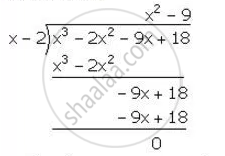Share

# Using the Factor Theorem, Show That: (X – 2) is a Factor of X3 – 2x2 – 9x + 18. Hence, Factorise the Expression X3 – 2x2 – 9x + 18 Completely. - Mathematics

Course

#### Question

Using the Factor Theorem, show that:

(x – 2) is a factor of x3 – 2x2 – 9x + 18. Hence, factorise the expression x3 – 2x2 – 9x + 18 completely.

#### Solution

Let f(x)=x^3-2x^2-9x+18

x-2=0 ⇒ x=2

∴ Remainder =f(2)

= (2)^3-2(2)^2-9(2)+18

=8-8-18+18

=0

Hence, (x-2) is a factor of f (x)

Now, we have:∴ x^3-2x^2-9x+18=(x-2)(x^2-9)=(x-2)(x+3)(x-3)

Is there an error in this question or solution?